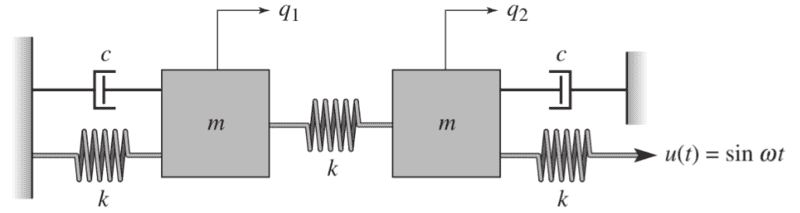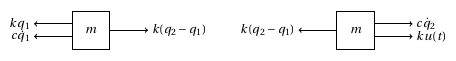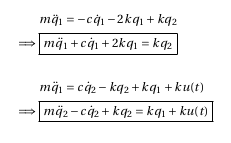# Equations of motion for a dual mass-spring-damper

## Homework Statement

We have been given the following mass-spring-damper diagram, and are asked to derive the equations of motion. The positions of the two masses are given as q1 and q2.## The Attempt at a Solution

I began by drawing free-body diagrams for each mass.Then I set up the equations using F_net = mq'' = sum of the forces acting on the mass.How does that look? I'm not very experienced at these, and I'm concerned that I've written some of the expressions incorrectly, and that I have some of the signs wrong as well. Thank you.